## Divergence

The divergence of a Vector Fieldis given by(1)

Define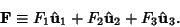(2)

Then in arbitrary orthogonal Curvilinear Coordinates,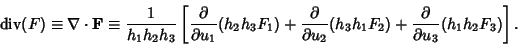(3)

If, then the field is said to be a Divergenceless Field. For divergence in individual coordinate systems, see Curvilinear Coordinates.(4)

The divergence of a Tensoris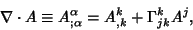(5)

whereis the Covariant Derivative and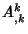is the Comma Derivative. Expanding the terms gives(6)

Arfken, G. Divergence,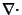.'' §1.7 in Mathematical Methods for Physicists, 3rd ed. Orlando, FL: Academic Press, pp. 37-42, 1985.